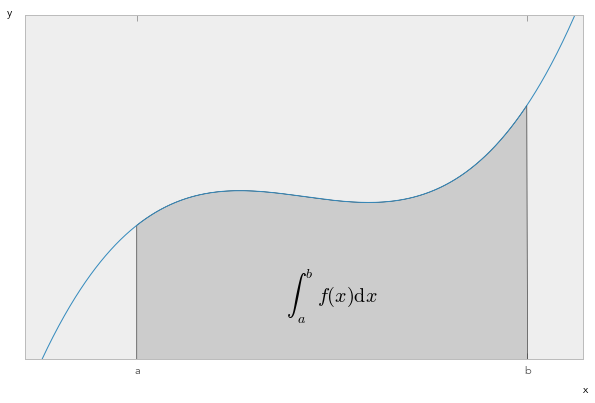# Running Code in the IPython Notebook¶

First and foremost, the IPython Notebook is an interactive environment for writing and running Python code.

## Code cells allow you to enter and run Python code¶

Run a code cell using Shift-Enter or pressing the "Play" button in the toolbar above:

In :
a = 10

In :
print(a)

10



## Managing the IPython Kernel¶

Code is run in a separate process called the IPython Kernel. The Kernel can be interrupted or restarted. Try running the following cell and then hit the "Stop" button in the toolbar above.

In :
import time
time.sleep(10)

---------------------------------------------------------------------------
KeyboardInterrupt                         Traceback (most recent call last)
<ipython-input-16-d7b436e260d5> in <module>()
1 import time
----> 2 time.sleep(10)

KeyboardInterrupt: 

If the Kernel dies you will be prompted to restart it. Here we call the low-level system libc.time routine with the wrong argument via ctypes to segfault the Python interpreter:

In [*]:
import sys
from ctypes import CDLL
# This will crash a Linux or Mac system; equivalent calls can be made on Windows
dll = 'dylib' if sys.platform == 'darwin' else 'so.6'
libc = CDLL("libc.%s" % dll)
libc.time(-1)  # BOOM!!


## All of the goodness of IPython works¶

Here are two system aliases:

In :
pwd

Out:
u'/Users/bgranger/Documents/Computation/IPython/code/ipython/examples/notebooks'

In :
ls

01_notebook_introduction.ipynb        Octave Magic.ipynb
Animations Using clear_output.ipynb   PyLab and Matplotlib.ipynb
Basic Output.ipynb                    R Magics.ipynb
Custom Display Logic.ipynb            Running Code.ipynb
Cython Magics.ipynb                   Script Magics.ipynb
Data Publication API.ipynb            SymPy Examples.ipynb
Display System.ipynb                  Trapezoid Rule.ipynb
JS Progress Bar.ipynb                 Typesetting Math Using MathJax.ipynb
Local Files.ipynb                     animation.m4v
Markdown Cells.ipynb                  python-logo.svg
Notebook Tour.ipynb



Any command line program can be run using ! with string interpolation from Python variables:

In []:
message = 'The IPython notebook is great!'
# note: the echo command does not run on Windows, it's a unix command.
!echo $message  Tab completion works: In : import numpy numpy.random.  Tab completion after ( brings up a tooltip with the docstring: In []: numpy.random.rand(  Adding ? opens the docstring in the pager below: In : magic?  Exceptions are formatted nicely: In : x = 1 y = 4 z = y/(1-x)  --------------------------------------------------------------------------- ZeroDivisionError Traceback (most recent call last) <ipython-input-15-dc39888fd1d2> in <module>() 1 x = 1 2 y = 4 ----> 3 z = y/(1-x) ZeroDivisionError: integer division or modulo by zero ## Working with external code¶ There are a number of ways of getting external code into code cells. Pasting code with >>> prompts works as expected: In : >>> the_world_is_flat = 1 >>> if the_world_is_flat: ... print("Be careful not to fall off!")  Be careful not to fall off!  The %load magic lets you load code from URLs or local files: In : %load?  In : %matplotlib inline  In : %load http://matplotlib.sourceforge.net/mpl_examples/pylab_examples/integral_demo.py  In : #!/usr/bin/env python # implement the example graphs/integral from pyx from pylab import * from matplotlib.patches import Polygon def func(x): return (x-3)*(x-5)*(x-7)+85 ax = subplot(111) a, b = 2, 9 # integral area x = arange(0, 10, 0.01) y = func(x) plot(x, y, linewidth=1) # make the shaded region ix = arange(a, b, 0.01) iy = func(ix) verts = [(a,0)] + list(zip(ix,iy)) + [(b,0)] poly = Polygon(verts, facecolor='0.8', edgecolor='k') ax.add_patch(poly) text(0.5 * (a + b), 30, r"$\int_a^b f(x)\mathrm{d}x\$", horizontalalignment='center',
fontsize=20)

axis([0,10, 0, 180])
figtext(0.9, 0.05, 'x')
figtext(0.1, 0.9, 'y')
ax.set_xticks((a,b))
ax.set_xticklabels(('a','b'))
ax.set_yticks([])
show()## Slide Show¶

In :
from IPython.display import HTML
HTML('<iframe src=http://www.slideviper.oquanta.info/tutorial/slideshow_tutorial_slides.html?useformat=mobile width=700 height=350></iframe>')

Out:
In []: